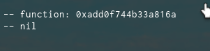# Issues with time converting

Hello scripters, I am working on my temp ban system and I am trying to convert the time to show how long is left on the user’s ban. I am having some issues with it.

My error:
“ReplicatedStorage.Common.Commands:96: attempt to perform arithmetic (add) on number and nil”

``````
-- My time converting that I use to set my ban time happened(what time the user got banned)

function convertTime(time)
local day = 86400
local hour = 3600
local minute = 60

local lengthNumber = tonumber(string.match(time, "%d+"))

local time = string.lower(time)
if string.find(time, "s") then
seconds = lengthNumber * 1
elseif string.find(time, "m") then
seconds = lengthNumber * minute
elseif string.find(time, "h") then
seconds = lengthNumber * hour
elseif string.find(time, "d") then
seconds = lengthNumber * day
end

print("Convert time")
return seconds
end

-- The time converter I use to check how long is left on the ban

function Format(Int)
return string.format("%02i", Int)
end

function convertToHMS(Seconds)
local day = 86400
local hour = 3600
local minute = 60

local Minutes = (Seconds - Seconds%60)/60
Seconds = Seconds - Minutes*60
local Hours = (Minutes - Minutes%60)/60
Minutes = Minutes - Hours*60
print(Minutes, Seconds, Hours)
return Format(math.abs(Hours)).."h"..Format(math.abs(Minutes)).."m"..Format(math.abs(Seconds)).."s"
end
``````

and here is the code I am trying to execute but errors.

``````
playerProfile.Data.BanTimeHappened = os.time()
playerProfile.Data.BanTime = convertTime(time)
local banTimeInFormat = convertToHMS(playerProfile.Data.BanTimeHappened + playerProfile.Data.BanTime - os.time())
print(tonumber(convertTime(os.time() - (playerProfile.Data.BanTimeHappened + playerProfile.Data.BanTime))))
``````

Which part of the script does it error in?

Right here, with my function not sure why.

You should see an error message in console with a little more information.

This is all there is in the output for errors.

`convertToHMS(playerProfile.Data.BanTimeHappened + playerProfile.Data.BanTime`

One of these is a number value the other is nil according to that error.

1 Like

I am aware but I can’t seem to find out why. I think it is my time converting

1 Like

Print both values, determine which is nil and which isn’t.

1 Like

BanTime appears to be the one to error any ideas?

``````playerProfile.Data.BanTime = convertTime(time)
``````

Something going wrong here then, check the function over.

Errors messages for operations in Luau are non-commutative so it’s easy to find out which data type is which. The first operand is a “number” and the second operand is “nil”.

To OP: What is the value of the variable `time` in `convertTime(time)`? It looks like the `convertTime` function returns nil.

Also avoid using time as a variable, it’s getting flagged as reserved.

1 Like

The time variable is the input text the user puts example: “1d” which my convertTime function is suppose to change to seconds

You to need to wrap it inside a call to “tonumber()” so that its type is correctly changed before performing any arithmetic operations on it.

Where? In the convert function I need it to be a string

What type of format does the value “time” typically use? Would help out a lot.``````print(time)
print(asda)
``````

I know you’re overriding time but you should still avoid using time. And let me know what format “time” is in when its being passed to the function.

So it takes like “1d” and will split the string looking for the number, if it is d then it does the amount of seconds in a day (86400) times the number provided, then returns it in seconds

Yeah, try a print on seconds before it’s returned.

``````function convertTime(time)
local day = 86400
local hour = 3600
local minute = 60
local seconds = 1
local lengthNumber = tonumber(string.match(time, "%d+"))

local time = string.lower(time)
if string.find(time, "s") then
seconds = lengthNumber * 1
elseif string.find(time, "m") then
seconds = lengthNumber * minute
elseif string.find(time, "h") then
seconds = lengthNumber * hour
elseif string.find(time, "d") then
seconds = lengthNumber * day
end

print("Convert time")
return seconds
end

print(convertTime("25d"))
``````

The function is working for me actually.